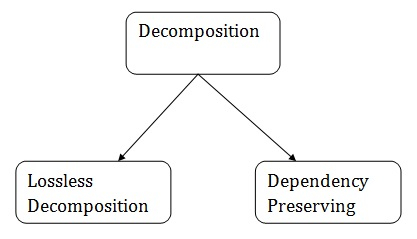# What is Decomposition in DBMS?

Decomposition means dividing a relation R into {R1, R2,......Rn}. It is dependency preserving and lossless.## Dependency preserving decomposition

Let R is decomposed into {R1, R2,....,Rn} with projected FD set {F1,F2,......Fn}. This decomposition is dependency preserving if F+ ={F1 U F2 U.........Fn}+.

## Example

Let the relation R{A,B,C,D,E} F:{AB->C, C->D, AB->D} R is decomposed to R1(A,B,C), R2(D,E). Prove decomposition is dependency preserving.

## Solution

F1={AB->C}

F2={C->D}

=> (F1 u F2) = {AB->C, C->D}

AB+ under (F1 U F2) = {A,B,C,D} => AB->D is under (F1 U F2)

F+ = (F1 U F2)+

=> Decomposition is dependency preserving.

## Decomposition is not preserving

Now consider another example where decomposition is not preserved.

Let the relation R{A,B,C,D,E,F,G,H,I,J} where F: {AB->C, A->DE, B->F, F->GH. D->IJ}

R is decomposed to R1(A,B,C,D), R2(D,E), R3(B,F), R4(F,G,H) AND R5(D,I,J). Check decomposition is dependency preserving or not.

## Solution

F1={AB->C}

F2={}

F3={B->F}

F4={F->GH}

F5={D->IJ}

=> (F1 U F2 U F3 U F4 U F5) = {AB->C, B->F, F->GH, D->IJ}

A+ under (F1 U F2 U F3 U F4 U F5) = {AB->C, B->F, F->GH, D->IJ}

=>A->DE is not under (F1 U F2 UF3 U F4 U F5)

=>F+ ≠ (F1 U F2 U F3 U F4 U F5)+

=> Decomposition is not dependency preserving.

## Lossless-join decomposition

It is a process in which a relation is decomposed into two or more relations. This property guarantees that the extra or less tuple generation problem does not occur, and no information is lost from the original relation during the decomposition. It is also known as non-additive join decomposition.

When the sub relations combine again then the new relation must be the same as the original relation was before decomposition.

Consider a relation R if we decomposed it into sub-parts relation R1 and relation R2.

The decomposition is lossless when it satisfies the following statement −

• If we union the sub–Relation R1 and R2 then it must contain all the attributes that are available in the original relation R before decomposition.

Intersections of R1 and R2 cannot be Null. The sub relation must contain a common attribute. The common attribute must contain unique data.

The common attribute must be a super key of sub relations either R1 or R2.

Here,

R = (A, B, C)

R1 = (A, B)

R2 = (B, C)

The relation R has three attributes A, B, and C. The relation R is decomposed into two relation R1 and R2. . R1 and R2 both have 2-2 attributes. The common attributes are B.

The Value in Column B must be unique. if it contains a duplicate value then the Lossless-join decomposition is not possible.

Draw a table of Relation R with Raw Data −

R (A, B, C)

ABC
122534
103609
124230

It decomposes into the two sub relations as follows −

R1 (A, B)

AB
1225
1036
1242

R2 (B, C)

BC
2534
3609
4230

We can now check the first condition for Lossless-join decomposition.

The union of sub relation R1 and R2 is the same as relation R.

R1U R2 = R

We get the following result −

ABC
122534
103609
124230

The relation is the same as the original relation R hence, the above decomposition is Lossless-join decomposition.